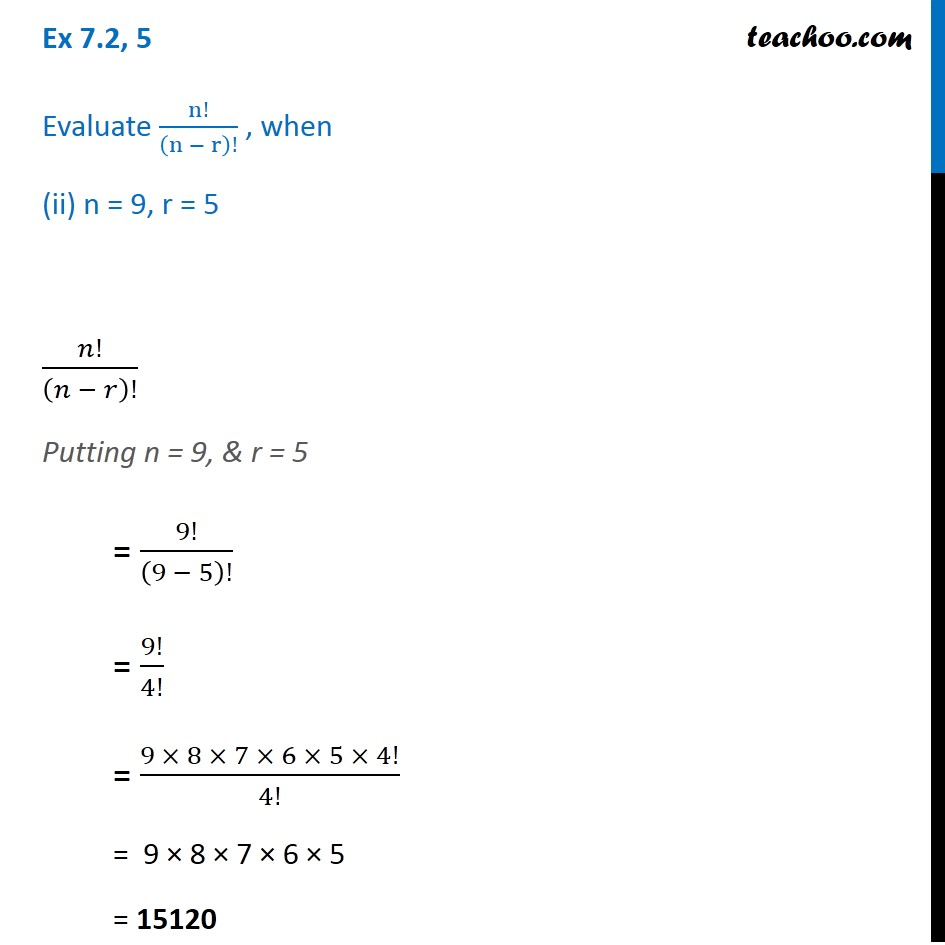1. Chapter 7 Class 11 Permutations and Combinations (Term 2)
2. Serial order wise
3. Ex 7.2

Transcript

Ex 7.2, 5 Evaluate n!/(n − r)! , when (ii) n = 9, r = 5 𝑛!/(𝑛 − 𝑟)! Putting n = 9, & r = 5 = 9!/(9 − 5)! = 9!/4! = (9 × 8 × 7 × 6 × 5 × 4!)/4! = 9 × 8 × 7 × 6 × 5 = 15120